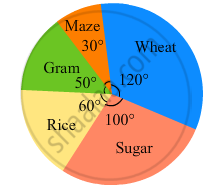Share

# The Pie-chart Given in the Following Shows the Annual Agricultural Production of an Indian State. If the Total Production of All the Commodities is 81000 Tonnes, Find the Production (In Tonnes) of - Mathematics

Course
ConceptIntroduction of Circle Graph Or Pie Chart

#### Question

The pie-chart given in the following shows the annual agricultural production of an Indian state. If the total production of all the commodities is 81000 tonnes, find the production (in tonnes) of
(i) Wheat
(ii) Sugar
(iii) Rice
(iv) Maize
(v) Gram#### Solution

(i) $\text{ Production of wheat }= \frac{\text{ Central angle for wheat }\times\text{ Total production }}{360^\circ}$
$= \frac{120^\circ \times 81000}{360^\circ} = 27000\text{ tonnes }$

(ii)$\text{ Production of sugar }= \frac{\text{ Central angle for sugar }\times\text{ Total production }}{360^\circ}$
$= \frac{10^\circ \times 81000}{360^\circ} = 22500\text{ tonnes }$

(iii) $\text{ Production of rice }= \frac{\text{ Central angle for Rice }\times\text{ Total production }}{360^\circ}$
$= \frac{60^\circ \times 81000}{360^\circ} = 13500\text{ tonnes }$

(iv) $\text{ Production of maize }= \frac{\text{ Central angle for maize }\times\text{ Total production }}{360^\circ}$
$= \frac{30^\circ \times 81000}{360^\circ} = 6750\text{ tonnes }$

(v) $\text{ Production of gram }= \frac{\text{ Central angle for Gram }\times\text{ Total production }}{360^\circ}$
$= \frac{120^\circ \times 81000}{360^\circ} = 11250\text{ tonnes }$

Is there an error in this question or solution?

#### APPEARS IN

RD Sharma Solution for Mathematics for Class 8 by R D Sharma (2019-2020 Session) (2017 to Current)
Chapter 25: Data Handling-III (Pictorial Representation of Data as Pie Charts or Circle Graphs)
Ex. 25.2 | Q: 2 | Page no. 21

#### Video TutorialsVIEW ALL 

Solution The Pie-chart Given in the Following Shows the Annual Agricultural Production of an Indian State. If the Total Production of All the Commodities is 81000 Tonnes, Find the Production (In Tonnes) of Concept: Introduction of Circle Graph Or Pie Chart.
S